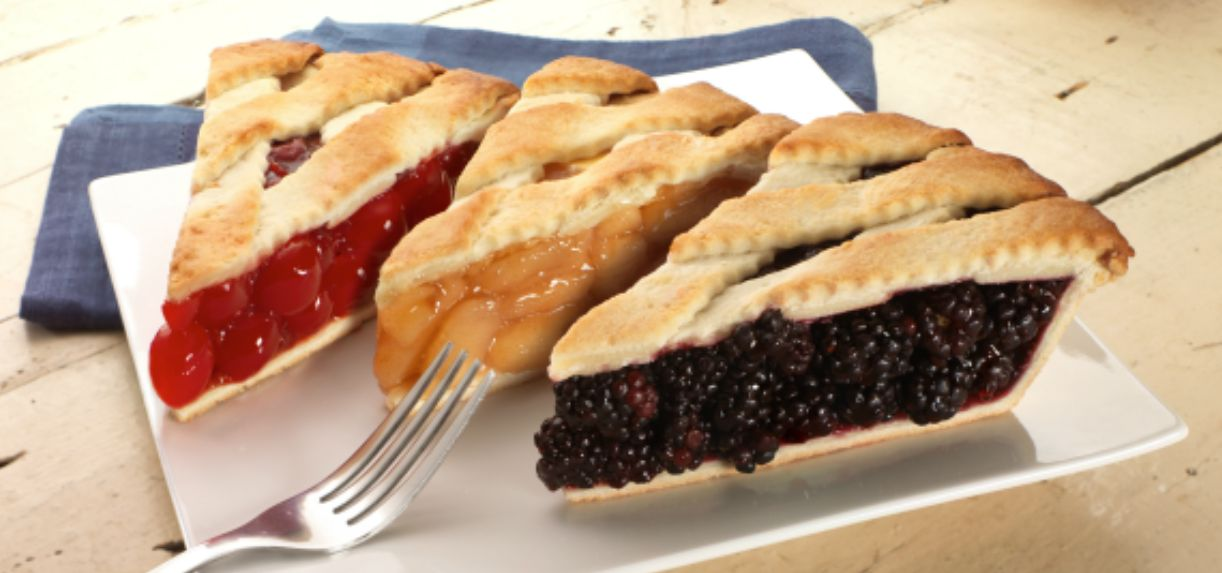# When a Mathematician Orders a Slice of Pie

Probability Level 4

A mathematician regularly orders a slice of pie for dessert at a restaurant where he meets for dinner with friends. On any day, the restaurant usually has available two out of three kinds of pies, Apple, Blackberry, and Cherry, which they make fresh on the premises.One evening, the waitress asks him what he would like to have, Blackberry or Cherry. The mathematician says he'll have the Blackberry. Then the waitress remembers, knowing that the mathematician often seems to prefer Apple over Blackberry, "Oh, by the way, we do also have Apple tonight. Would you like to have that?" The mathematician says,

"Well, in that case, I'll have the [...]."

As the mathematician explains to his friends, different people make the pies on different days at random, of variable quality. Over time, he's come up with a scoring system as follows:

Over $30$ days that any of the pies are made,

• the Apple scores $\color{#D61F06} \large 3$ on all $30$ days;
• the Blackberry scores $\color{#D61F06} \large 2$ on $18$ days, $\color{#D61F06} \large 4$ on $6$ days, and $\color{#D61F06} \large 6$ on $6$ days;
• the Cherry scores $\color{#D61F06} \large 1$ on $16$ days, $\color{#D61F06} \large 5$ on $14$ days.

So, on any given day, he always orders the pie that is most likely to have the highest score among all that is available on that day. (See note.)

What pie did the mathematician order that evening?

Note: Not the highest expected score, but which pie is likely to have the highest score on that day. Imagine tossing two or three $30$-sided dice and checking to see which die comes up with the highest number, which have numbers on the faces as follows:

• Apple die: $3$ on all $30$ faces
• Blackberry die: $2$ on $18$ faces, $4$ on $6$ faces, $6$ on $6$ faces
• Cherry die: $1$ on $16$ faces, $5$ on $14$ faces

Whichever die comes up with the highest number wins. Whether there's $2$ or $3$ dice being played, the mathematician goes with the one that is most likely to win. $30$ sided dice are possible in which any side has equal probability of coming up, based on the Rhombic Triacontahedron

Comments about the solution and the mathematician's reasoning would be most welcome.

×